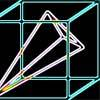#### You may also likeA box of size a cm by b cm by c cm is to be wrapped with a square piece of wrapping paper. Without cutting the paper what is the smallest square this can be?### Plane to See

P is the midpoint of an edge of a cube and Q divides another edge in the ratio 1 to 4. Find the ratio of the volumes of the two pieces of the cube cut by a plane through PQ and a vertex.### Four Points on a Cube

What is the surface area of the tetrahedron with one vertex at O the vertex of a unit cube and the other vertices at the centres of the faces of the cube not containing O?

# Interpenetrating Solids

##### Age 16 to 18 Challenge Level:

For the first part of this problem try to view the cubes from above to turn it into a simpler 2D problem to begin with.

The second part of this problem will be greatly simplfied if you work with a physical model of a cube, preferably wire framed which you can see through (such as with polydron) and the bigger the better. You may wish to attempt to create frames using wires or straws to help to understand the problem.

Instead of trying to visualise the entire solid 3 dimensional cube, you may like to consider the cube as defined by its 8 corners. If you work out where the corners go then the rest of the cube will follow by joining up with straight lines and planes.Visualising the objects by looking directly at one of the original faces will help you to understand the problem.

Finally, don't forget that if you can work out the view of the interpenetrated object in the direction of one of the faces, then the view in the direction of the other faces may follow by symmetry.